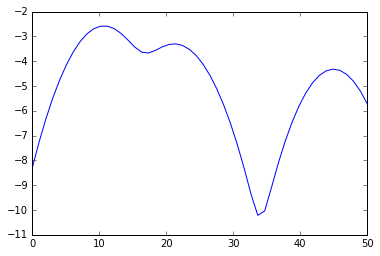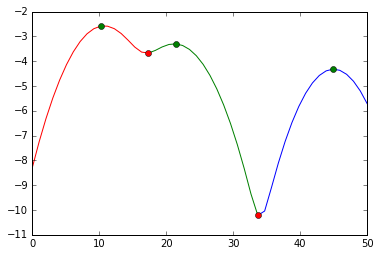2 views

I need to cluster a simple univariate data set into a preset number of clusters. Technically it would closer to binning or sorting the data since it is the only 1D, but my boss is calling it clustering, so I'm going to stick to that name. The current method used by the system I'm on is K-means, but that seems like overkill.

Is there a better way of performing this task?

Answers to some other posts are mentioning KDE (Kernel Density Estimation), but that is a density estimation method, how would that work?

I see how KDE returns a density, but how do I tell it to split the data into bins?

How do I have a fixed number of bins independent of the data (that's one of my requirements) ?

More specifically, how would one pull this off using scikit learn?

My input file looks like:

str ID     sls

1           10

2           11

3            9

4           23

5           21

6           11

7           45

8           20

9           11

10          12

I want to group the SLS number into clusters or bins, such that:

Cluster 1: [10 11 9 11 11 12]

Cluster 2: [23 21 20]

Cluster 3: 

And my output file will look like:

str ID     sls Cluster ID  Cluster centroid

1        10 1               10.66

2        11 1               10.66

3         9 1               10.66

4        23 2               21.33

5        21 2               21.33

6        11 1               10.66

7        45 3               45

8        20 2               21.33

9        11 1               10.66

10       12 1               10.66

by (33.1k points)

%matplotlib inline

from numpy import array, linspace

from sklearn.neighbors.kde import KernelDensity

from matplotlib.pyplot import plot

a = array([10,11,9,23,21,11,45,20,11,12]).reshape(-1, 1)

kde = KernelDensity(kernel='gaussian', bandwidth=3).fit(a)

s = linspace(0,50)

e = kde.score_samples(s.reshape(-1,1))

plot(s, e)from scipy.signal import argrelextrema

mi, ma = argrelextrema(e, np.less), argrelextrema(e, np.greater)

print "Minima:", s[mi]

print "Maxima:", s[ma]

> Minima: [ 17.34693878  33.67346939]

> Maxima: [ 10.20408163  21.42857143 44.89795918]

print a[a < mi], a[(a >= mi) * (a <= mi)], a[a >= mi]

> [10 11  9 11 11 12] [23 21 20] 

You can also do this split:

plot(s[:mi+1], e[:mi+1], 'r',

s[mi:mi+1], e[mi:mi+1], 'g',

s[mi:], e[mi:], 'b',

s[ma], e[ma], 'go',

s[mi], e[mi], 'ro')Here we cut at the red markers. The green markers are our best estimates for the cluster centers.